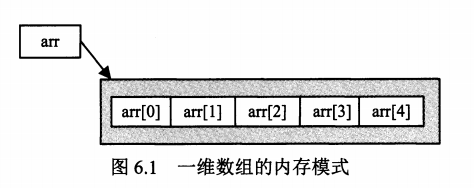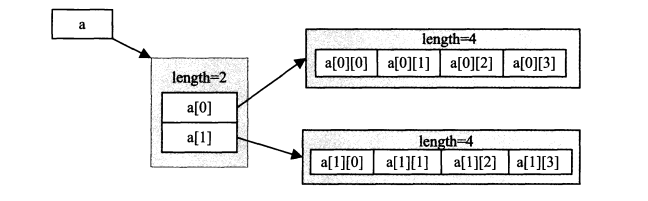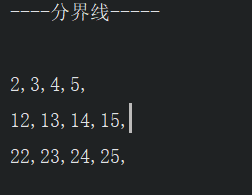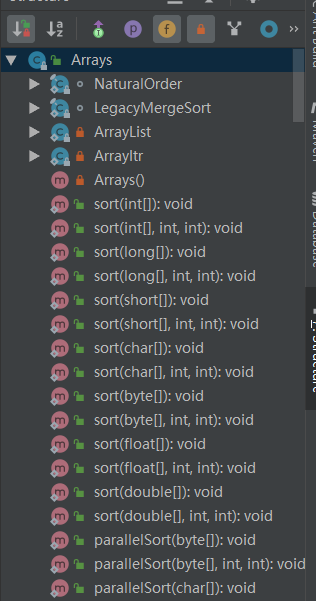## [java初探05]__数组的简单认识及Arrays类的常用方法 转

o
osc_gu9d45li

• 关于一维数组与二维数组

• 数组的创建于初始化

• 一维数组的创建

``````int a[]; // 声明int型数组
int[] b=new int; // 声明的同时直接分配内存大小
a = new int ; //为其分配内存大小
``````• 一维数组的初始化 在前面我们知道了,数组的每个元素的表示,都是以数组的下标来表示的.那么我们想要对数组中的元素进行操作的化,必然要从数组的下标入手.

• 静态初始化 我们可以直接在创建数组的时候使用{}直接为数组初始化赋值,实现一步直接创建并初始化数组赋初值.这样就省略了分配内存的步骤.

``````int[] b={1,2,3,4};
``````
• 动态初始化 我们也可以直接通过数组的下标来直接对数组中的某个元素赋值初始化,称作动态初始化,动态初始化前,我们需要先为数组分配内存空间.
``````  int[] a;
a =new int;
a=1;
a=2;
a=3;
``````

• 二维数组的创建与初始化

``````
int arr[][];
int[][] arr2;
arr2 = new int;

int[][] c={{2,3},{12,13},{22,23}};
System.out.println(c);
int[][] d=new int;
d=1;
d=2;
d=3;
d=4;
d=11;
d=12;
d=13;
d=14;

``````• 数组操作

• 遍历数组 通过for循环遍历数组,将数组内容全部输出.一维数组的遍历十分简单:

``````int[] a={1,2,3,4,5};
for(int i=0;i<a.length;i++){
System.out.print(a[i]+",");
}
``````
• 二维数组的遍历

``````  // 二维数组的遍历
int[][] b={{2,3,4,5},{12,13,14,15},{22,23,24,25}};
for(int i=0;i<b.length;i++){ // 通过两个for循环遍历二维数组,外层循环遍历列的下标,
for (int j=0;j<b[i].length;j++){  // 内存循环循环遍历行的下标.
System.out.print(b[i][j]+","); //循环输出对应下标的元素.遍历出整个二维数组.
}
System.out.println(); // 输出换行(每行数据输出红后换行.)
}
``````• 替换数组元素

Arrays类中给我们提供了fill方法用来填充后替换数组中的元素.其重载有多种方法提供不同形式的替换方式.fill(int[],int)方法可以将指定值替换(填充)到指定数组的所有元素中.fill(int[],int ,int,int)方法将指定值替换到指定范围的元素中,前两个int参数指定数组下标范围.

``````  //填充数组
Arrays.fill(c,6);
System.out.println(Arrays.toString(c));
Arrays.fill(c,3,7,10);
System.out.println(Arrays.toString(c));
``````
• 数组排序 Arrays类中还提供了排序方法sort()其也重载有多个方法.最简单的排序方法sort(object)参数及需要排序的数组.
`````` Arrays.sort(c);
System.out.println(Arrays.toString(c));
``````
• 复制数组 Arrays类也提供有copyOf()方法和copyOfRange()方法用来将一个数组复制到另一个新数组中.copyOfrange()方法可以指定copy范围.
``````//copy数组
int[] d=Arrays.copyOf(c,12);
int[] e=Arrays.copyOfRange(c,3,7);
System.out.println(Arrays.toString(d));
System.out.println(Arrays.toString(e));
``````
• 数组查询 Arrays类的binarySearch()方法,可使用二分法来搜索指定数组.其也提供有多种重载方式,可以在指定范围搜索数组.返回值为数组所在位置(不是返回其下标).
``````//数组查询
//数组查询
System.out.println(Arrays.binarySearch(c,10));
System.out.println(Arrays.binarySearch(c,5,7,10));

``````• 源码
``````import java.sql.SQLOutput;
import java.util.Arrays;

/**
* @outhor xiaoshe
* @date 2019/4/5  - @time 20:40
* 数组
*/
public class Sty_Array {

public static void main(String[] args) {
Sty_Array sty_array = new Sty_Array();
sty_array.create_array();
sty_array.TraverArrays();

}

// 两种数组的创建,我们可以将代表数组的符号[]加给变量或者数据类型关键字.

public void create_array(){
// 一维数组
int[] a;
a =new int;
a=1;
a=3;
System.out.println(a);
System.out.println(Arrays.toString(a));
System.out.println(a.length);
char[] b ={'a','b','c','d'};
System.out.println(Arrays.toString(b));
// 二维数组
int[][] c={{2,3},{12,13},{22,23}};
System.out.println(c);
int[][] d=new int;
d=1;
d=4;
d=11;
}

// 数组操作
public void TraverArrays(){
//一维数组的遍历
int[] a={1,2,3,4,5};
int[] c={3,6,2,4,1,7,9,8,0};
for(int i=0;i<a.length;i++){
System.out.print(a[i]+",");
}
System.out.println("\n----分界线-----");
// 二维数组的遍历
int[][] b={{2,3,4,5},{12,13,14,15},{22,23,24,25}};
for(int i=0;i<b.length;i++){ // 通过两个for循环遍历二维数组,外层循环遍历列的下标,
for (int j=0;j<b[i].length;j++){  // 内存循环循环遍历行的下标.
System.out.print(b[i][j]+","); //循环输出对应下标的元素.遍历出整个二维数组.
}
System.out.println(); // 输出换行(每行数据输出红后换行.)
}
//数组排序
Arrays.sort(c);
System.out.println(Arrays.toString(c));
//copy数组
int[] d=Arrays.copyOf(c,12);
int[] e=Arrays.copyOfRange(c,3,7);
System.out.println(Arrays.toString(d));
System.out.println(Arrays.toString(e));
//填充数组
Arrays.fill(c,6);
System.out.println(Arrays.toString(c));
Arrays.fill(c,3,7,10);
System.out.println(Arrays.toString(c));
//数组查询
System.out.println(Arrays.binarySearch(c,10));
System.out.println(Arrays.binarySearch(c,5,7,10));
}
}

``````

o

### osc_gu9d45li

1、Java入门篇 1.1 基础入门和面向对象 1.1.1 编程基础  Java语言的基本认识  类和对象  类的结构和创建对象  包和访问权限修饰符  利用private来封装  Java的数据类...

osc_t2w0zvsf
2019/04/29
0
0
【《实战java虚拟机》学习笔记】07. 分析java堆（二）：MAT对OQL的支持

Funcy1122
2019/11/17
0
0
Java 零基础入门 2019版

2019/06/24
2
0

05/12
12
0
java面

osc_yuku1blu
2019/01/07
3
0

IamOkay
15分钟前
31
0

25分钟前
0
0

① 进程与线程 程序、进程、线程、协程的概念 程序： 用某种语言编写的一组指令的集合，即指一段静态的代码； 进程：简单地说就是一个正在执行的程序或应用，是资源分配的最小单位； 线程：线...

Arno_pei
38分钟前
0
0
08VulKan——描述符布局、缓冲、描述符池和描述符集

49分钟前
7
0

chipo
52分钟前
18
0